# 通过阶乘的例子,练习在JavaScript, Scala和ABAP里实现尾递归(Tail Recursion)

Before we start to research tail recursion, let’s first have a look at the normal recursion.

A simple factorial implementation by recursion:

function factorial(n){
if(n ===1) {
return 1;
}
return n *factorial(n -1);
}


Let N = 5, see how new stack frame is created for each time of recursive call: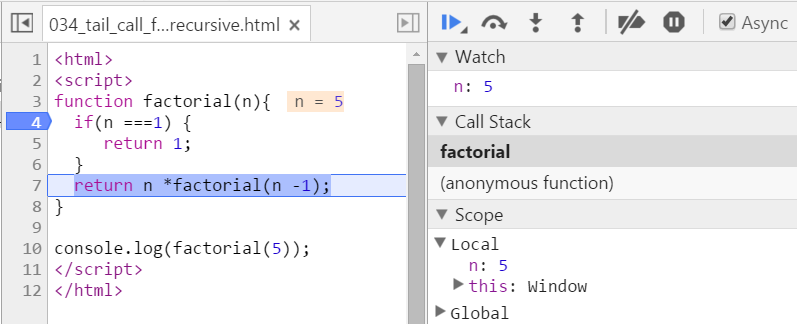We have two stack frames now, one stores the context when n = 5, and the topmost one for current calculation: n = 4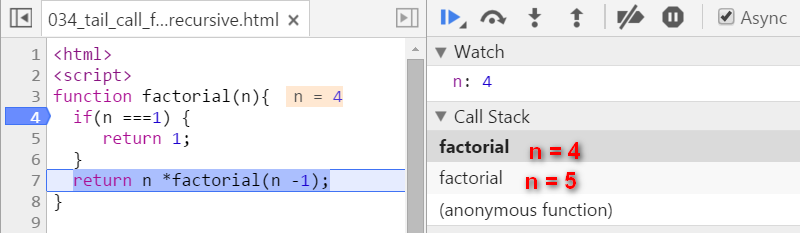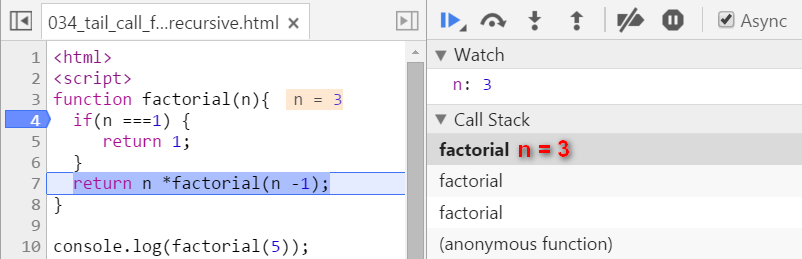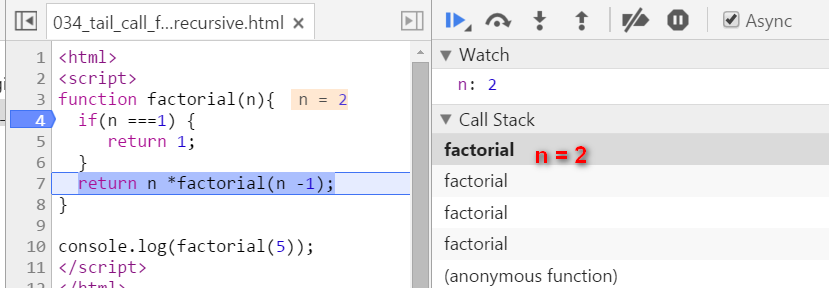Now since n equals to 1, we stop recursion. The current stack frame ( n = 1 ) will be poped up, the frame under it will be activated and become the topmost frame, with calculated result 1 passed into.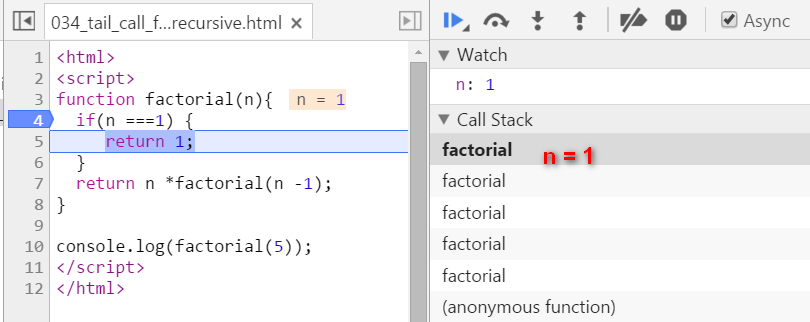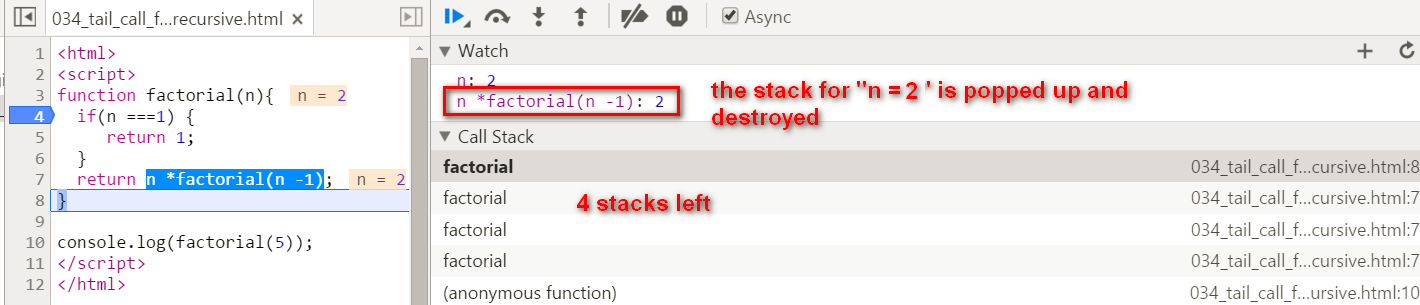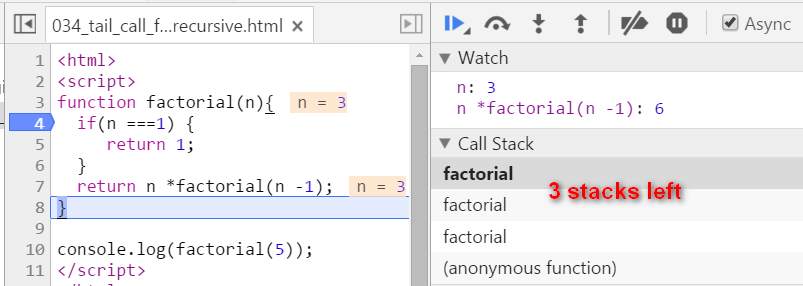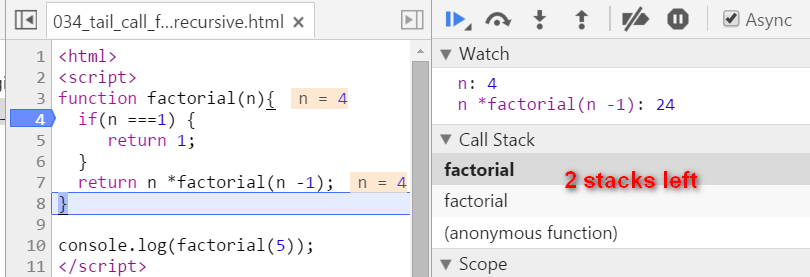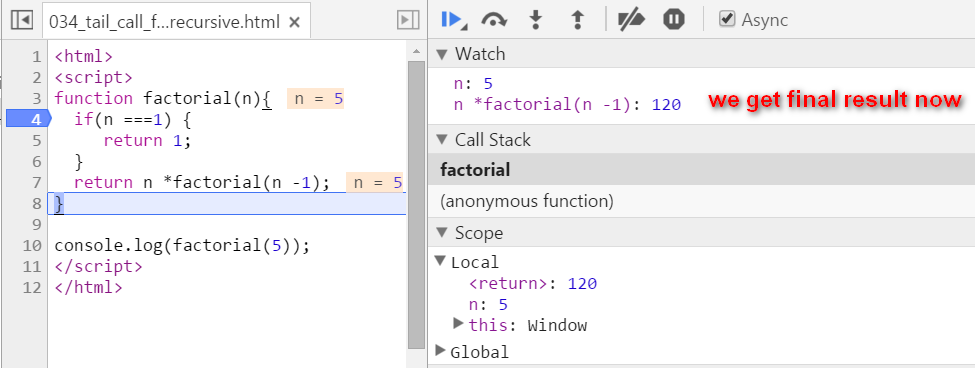key note for normal recursion: during recursion, every generated stack frame is needed and could not e destroyed until the final result is calculated. The calculation is actually not started until the recursion reaches the end ( the condition n === 1 fulfills ). If N is a big integer, it will lead to huge number of stack frames and finally the “stack overflow” or “out of memory” is inevitable.

# tail recursion

Source code below:

function tailFactorial(n, total) {
if(n ===1)
return tailFactorial(n -1, n * total);
}
function factorial2(n) {
return tailFactorial(n,1);
}


There are two biggest differences compared with normal recursion:

(1) A new internal function tailFactorial is introduced here.

(2) The calculation is actually now spread within every recursive stack frame. Each frame finishes one part of calculation and pass the current result to the next frame. Once the current stack frame finishes its task, it is actually not needed any more. And thus for example the model browser can then do some optimization on those useless stack frames.

Observe the stack frame for tail recursion step by step: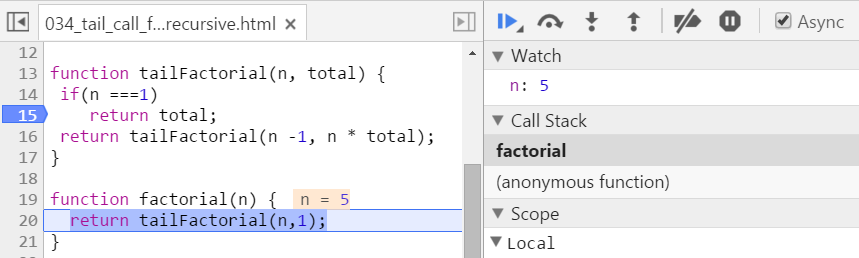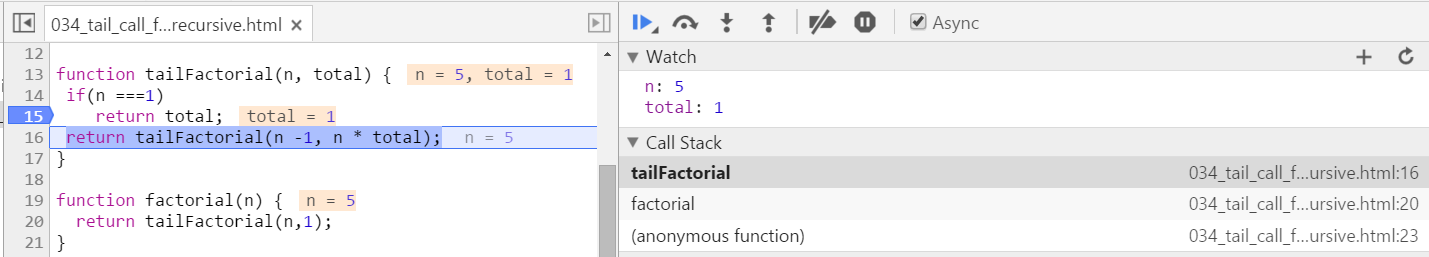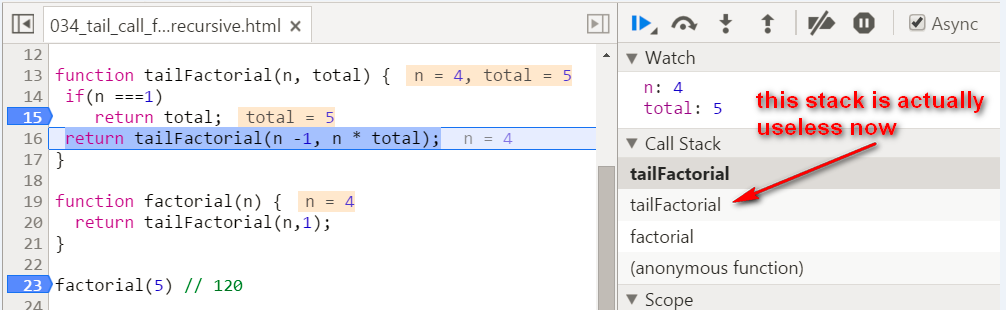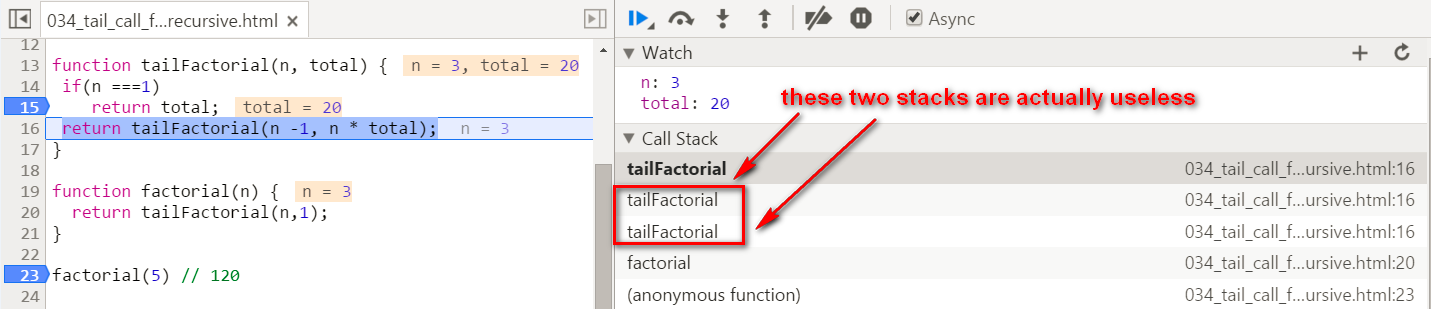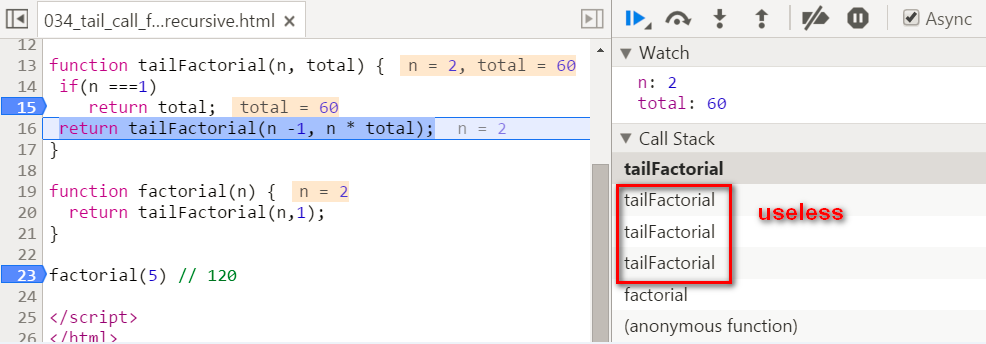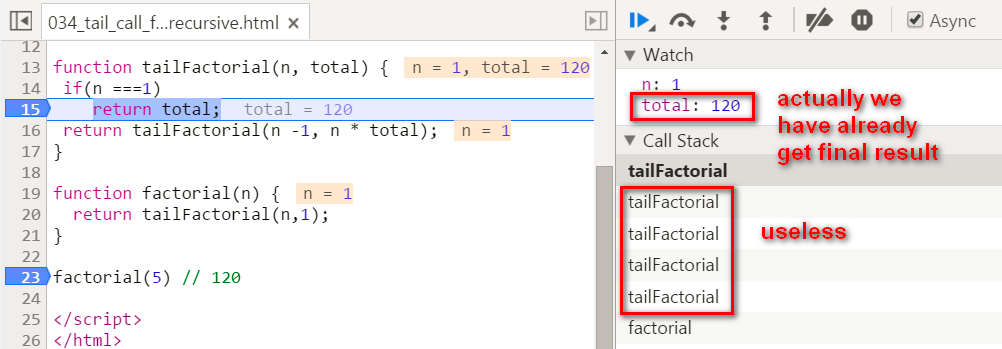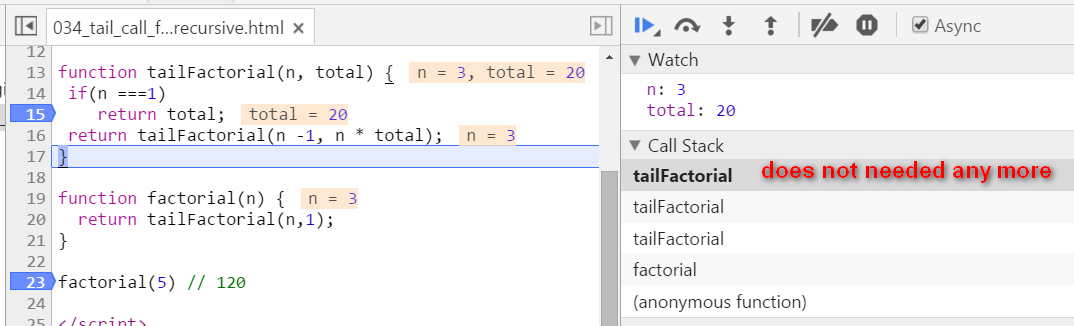stack popped up: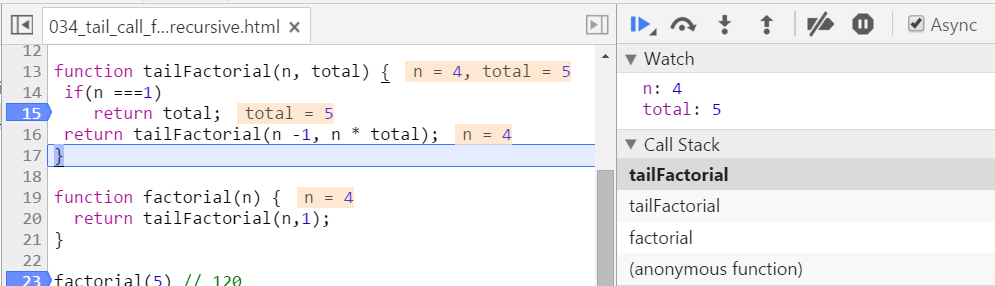When N = 20, the tail recursion has a far better performance than the normal recursion: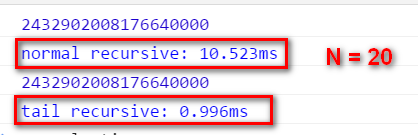# Update 2016-01-11

Tail recursion implementation via Scala: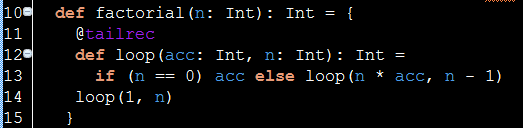The interesting thing is, after the Scala code is compiled into Java Byte code, compiler will eliminate the recursion automatically: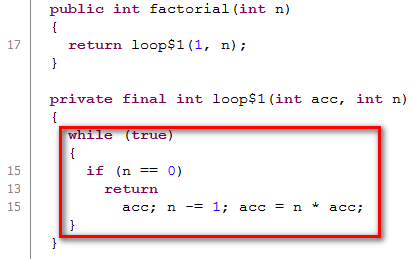# Tail Recursion in ABAP

First this is the normal recursion:

REPORT zrecursion.

START-OF-SELECTION.

DATA: lv_result TYPE int4.

PERFORM fac USING 6 CHANGING lv_result.

WRITE: / lv_result.

FORM fac USING iv_n TYPE int4 CHANGING cv_result TYPE int4.
DATA: lv_n TYPE i.

cv_result = lv_n = iv_n.
lv_n = lv_n - 1.
IF lv_n > 1.
PERFORM fac USING lv_n CHANGING cv_result.
ENDIF.
IF lv_n = 1.
cv_result = cv_result * lv_n.
ELSE.
cv_result = cv_result * iv_n.
ENDIF.
ENDFORM.


And here comes tail recursion version:

REPORT ztail.

START-OF-SELECTION.

DATA: lv_result TYPE int4.

PERFORM fac USING 5 1 CHANGING lv_result.

WRITE: / lv_result.

FORM fac USING iv_n TYPE int4 iv_acc TYPE int4 CHANGING cv_result TYPE int4.
DATA: lv_n          TYPE i,
lv_accumulate TYPE i.

IF iv_n < 1.
cv_result = 1.
ELSEIF iv_n = 1.
cv_result = iv_acc * iv_n.
ELSEIF iv_n > 1.
lv_n = iv_n - 1.
lv_accumulate = iv_acc * iv_n.
PERFORM fac USING lv_n lv_accumulate CHANGING cv_result.
ENDIF.
ENDFORM.04-173140

#### JavaScript中实现一个整数阶乘的方法

05-011万+

#### javascript实现n阶乘的2个方法01-3031

#### 尾递归(Tail recursion / Tail call)

03-1652

#### 用尾递归(tailRecursive)实现的阶乘-JavaScript版本

01-1290

#### 使用ABAP REDUCE关键字编写的一个非递归阶乘实现

08-2421

#### ABAP Netweaver体内的那些寄生式编程语言

02-14106

#### ABAP自定义功能函数

10-2985

#### BOM查找上阶层物料函数

10-1523

#### 递归的用法

03-29235

#### SAP自开发程序

04-18241

#### api文档、packpage的概念、生成自己项目的api文档

06-231355

#### oracle:优化2----使用hints,加速生成执行计划

05-1129

#### case、while、until、select循环

09-034062

#### 数值溢出（arithmetic overflow）问题与解决方案

02-2737

#### 编程语言大简介（基于《2011程序员薪资调查报告》）

03-301501

#### 调用程序与子程序之间进行数据传递的方式

05-2015

#### 关于《博客园精华集》第一轮收录情况（仅针对2008年5月19日起的一些文章的记录）...

09-031万+

#### 华为笔试题©️2020 CSDN 皮肤主题: 深蓝海洋 设计师: CSDN官方博客点击重新获取扫码支付1.余额是钱包充值的虚拟货币，按照1:1的比例进行支付金额的抵扣。
2.余额无法直接购买下载，可以购买VIP、C币套餐、付费专栏及课程。余额充值